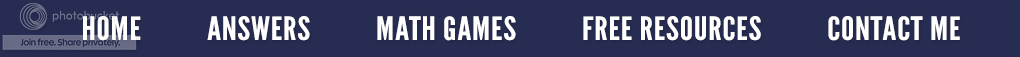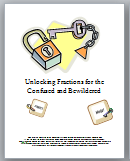### The Power of Math Tricks

Math tricks will never make you a great mathematician, but in the eyes of some, you can be a fantastic math-a-magician. My college students love it when I show them a trick they can then take home to amaze and impress their peers, parents, children or the best yet - their spouses.

Remember when I demonstrated how to easily multiply by 11 in the post The Eleventh Hour?  Or how about the trick of multiplying by 12 in Quick Times?   Here is a new one I recently showed my students.

First, let's look at a problem where a number is divided by the decimal .25

The above example is really 9 x 4 which is 36, but why is this true?  Hopefully your students know that .25 is equivalent to 1/4; so this problem can be reworded as  9 divided by 1/4.  As seen below, when dividing a whole number by a fraction, we find the reciprocal of 1/4 and then multiply which gives us the answer of 36.

Based on the sample above, anytime a problem requires dividing by .25, simply multiply by four to get the correct answer.  Try these without using a calculator or paper and pencil.

﻿
﻿
Instead of using the reciprocal to divide fractions, I teach my students that this is the "cross" method. Simply look at the original problem and cross multiply as seen in the illustration below.
﻿Fractions for the Confused and Bewildered

First multiply the bottom right denominator with the top left numerator. (4 x 9)  Next multiply the bottom left denominator with the top right numerator, (1 x 1) and you get an answer of 36.  When doing the fractions this way, there is no confusion on the students' part about which fraction to invert. If you would like a more details on how to divide fractions this way, go to the post entitled: Don't Flip!

If you are interested in other alternative ways to teach the four operations of fractions, you can check out the resource on your right.

By the way, the answers to the above problems are  a) 24    b) 80    c) 72    d) 380.    How did you do?

### Palindromic Words and Numbers

I was getting ready to pay for my meal at a buffet when I noticed the cashier's name tag.  It read "Asa" to which I replied, "Your name is a palindrome!"  The cashier just stared at me in disbelief.  I explained that a palindrome was letters that read the same backwards as forwards. Because you could read his name forwards and backwards, it qualified as a palindrome.  He replied that he remembered a math teacher talking about those because of patterns (I love that math teacher), and he remembered the phrase "race car" was a palindrome.  We then began sharing palindromes that we knew such as radar, level and madam while my family waited impatiently in line. (Sometimes they have little patience with my math conversations.)

The word palindrome is derived from the Greek word palíndromos, which means "running back again". A palindrome can be a word, phrase or sentence which reads the same in both directions such as: "Eva, can I stab bats in a cave?" or "Was it a car or a cat I saw?" or "Rats live on no evil star."

But did you know there are also palindromic numbers?  A palindromic number is a number whose digits are the same if read in both directions (as seen on your left).   Whereas "1234" is not a palindromic number, because backwards it is "4321" which is not the same.

Suppose a person starts with the number one and lists the palindromic numbers in order: 11, 22, 33, 44, 55...etc.  Can you continue the list?

Did you notice that palindromic numbers are symmetrical?  Look carefully at the 17371 shown above.  It is symmetrical (when a figure can be folded along a line so the two halves match perfectly) on either side of the three whether read left to right or vice versa.

Palindromic numbers are very simple to generate from other numbers with the help of addition.

Try this:

1. Write down any number that has more than one digit. I will use 47.
2. Write down that number in reverse beneath the first number. (See illustration below.)
3. Add the two numbers together. (121)
4. The sum of 121 is undeniably a palindrome.

Try an easy number first, such as 18.  At times you will need to use the first addition answer and repeat the process of reversing and adding. You will almost always get a palindrome answer within six steps.  Try one of these numbers 68 or 79.  Be careful because if you pick a number greater than 89, arriving at the palindromic answer will take more steps, but it will still work.  (See the two examples below.)

But don't try 196!  In fact, avoid it like the plague!   A computer has already gone through several thousand stages, and it still hasn't come up with a palindromic answer!

Example #1:

Reverse 75 which makes 57.

Add 75 and 57 and you get 132.  The answer 132 is not a palindrome.

SO reverse 132, and it becomes 231.

Add 132 and 231, and the answer is 363. Since 363 is a palindrome, we are done!

Example #2:
Begin with 255.

Reverse 255 to get 552.

Add 255 and 552.  The answer is 807 which is not a palindrome.

SO reverse 807 to get 708.

Add 807 and 708.  The answer of 5151 is not a palindrome.

SO reverse 1515 to get 5151.

Add 1515 and 5151 which is 6666.  This is a palindrome; so, we are done!